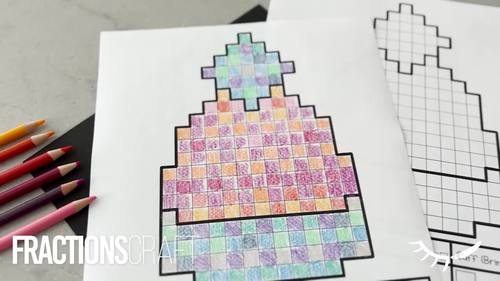# Fractions to Decimals Worksheet | How to Convert Decimal to Fraction

Rated 5 out of 5, based on 2 reviews
2 Ratings;
4th - 6th, Homeschool
Subjects
Resource Type
Standards
Formats Included
• Zip
Pages
16 pages
Report this resource to TPT

### Description

Looking for an easy converting fractions to decimals worksheet? This decimals to fractions worksheet is a fun way to merge art and math.

*It is helpful if students are already familiar with decimals and fractions first. This decimals worksheet activity is a great way for students to apply their knowledge of how to convert decimals to fractions in a new context.

Converting Decimals & Fractions 2 Directions!

How it the Decimal and Fraction Worksheets Work?

The toque is divided into three segments. The pompom is made up of 25 squares, the body of the toque is 100 squares and the brim is 50 squares.

This provides varying degrees of challenge for calculating decimals and fractions.

*It is helpful if students have some prior understanding of decimals and equivalent fractions before doing this activity.

Check out the video preview to see the decimals worksheets in action!

Templates vary depending on learning objectives:

Pg. 6: Toque image only

Pgs. 7-8: Blank template with boxes

Pgs. 9-14: Blank templates with spots for decimals. Some show value of the boxes (0.01, 0.02, and 0.04).

Pgs. 15-16: Blank template with fraction lines

Pgs. 17-19: Given fractions to convert to decimals

Pgs. 20-22: Given decimals to convert to fractions.

Included:

-Printable decimals to fraction worksheet

-Video Instructions of how to complete the craft

-Visual Instructions

Connect with Me:

I would also LOVE to see how you use them in your classroom! Feel free to tag me or send me a message if you have any questions.

New products are posted at a discount for the first 48 hours! Follow me to know about sales and new product releases.

instagram: @draw.calm

Total Pages
16 pages
N/A
Teaching Duration
1 hour
Report this resource to TPT
Reported resources will be reviewed by our team. Report this resource to let us know if this resource violates TPT’s content guidelines.

### Standards

to see state-specific standards (only available in the US).
Explain why a fraction 𝘢/𝘣 is equivalent to a fraction (𝘯 × 𝘢)/(𝘯 × 𝘣) by using visual fraction models, with attention to how the number and size of the parts differ even though the two fractions themselves are the same size. Use this principle to recognize and generate equivalent fractions.
Decompose a fraction into a sum of fractions with the same denominator in more than one way, recording each decomposition by an equation. Justify decompositions, e.g., by using a visual fraction model. Examples: 3/8 = 1/8 + 1/8 + 1/8; 3/8 = 1/8 + 2/8; 2 1/8 = 1 + 1 + 1/8 = 8/8 + 8/8 + 1/8.
Add and subtract fractions with unlike denominators (including mixed numbers) by replacing given fractions with equivalent fractions in such a way as to produce an equivalent sum or difference of fractions with like denominators. For example, 2/3 + 5/4 = 8/12 + 15/12 = 23/12. (In general, 𝘢/𝘣 + 𝘤/𝘥 = (𝘢𝘥 + 𝘣𝘤)/𝘣𝘥.)
Express a fraction with denominator 10 as an equivalent fraction with denominator 100, and use this technique to add two fractions with respective denominators 10 and 100. For example, express 3/10 as 30/100, and add 3/10 + 4/100 = 34/100.
Use decimal notation for fractions with denominators 10 or 100. For example, rewrite 0.62 as 62/100; describe a length as 0.62 meters; locate 0.62 on a number line diagram.